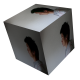# PCA and rggobi

```#generate row names
rname <- c(1:10000)
#generate column names
cname <- c(rep("control",20),rep("test",20))
#initialise array with 10,000 rows and 40 columns
data <- array(0,dim=c(10000,40),dimnames=list(rname,cname))
for (i in 1:nrow(data)){
x_mean <- sample(1:10000,1)
x_sd <- sample(1:1000,1)
y_mean <- sample(1:10000,1)
y_sd <- sample(1:1000,1)
x <- rnorm(20,mean=x_mean,sd=x_sd)
y <- rnorm(20,mean=y_mean,sd=y_sd)
for (j in 1:20){
data[i,j] <- x[j]
}
for (k in 1:20){
k_1 <- 20 + k
data[i,k_1] <- y[k]
}
}
data.pca <- prcomp(data)
x <- data.pca\$rotation[,"PC1"]
y <- data.pca\$rotation[,"PC2"]
xy <- data.frame(x,y)
library(rggobi)
g <- ggobi(xy)
```I labelled only two samples since the text would overlap. Samples 1 to 20 are located near the 16 and samples 21 to 40 near the 37, as expected.1.Andy Kwok says:
1.Davo says: